# 十种常见排序算法（转）

http://blog.csdn.net/k346k346/article/details/50791102

# 1.常见算法分类

（1）非线性时间比较类排序：交换类排序（快速排序和冒泡排序）、插入类排序（简单插入排序和希尔排序）、选择类排序（简单选择排序和堆排序）、归并排序（二路归并排序和多路归并排序）；

（2）线性时间非比较类排序：计数排序、基数排序和桶排序。

（1）在比较类排序中，归并排序号称最快，其次是快速排序和堆排序，两者不相伯仲，但是有一点需要注意，数据初始排序状态对堆排序不会产生太大的影响，而快速排序却恰恰相反。

（2）线性时间非比较类排序一般要优于非线性时间比较类排序，但前者对待排序元素的要求较为严格，比如计数排序要求待排序数的最大值不能太大，桶排序要求元素按照hash分桶后桶内元素的数量要均匀。线性时间非比较类排序的典型特点是以空间换时间。

# 2.算法描述与实现

## 2.1交换类排序

### 2.1.1冒泡排序

（1）从数组中第一个数开始，依次与下一个数比较并次交换比自己小的数，直到最后一个数。如果发生交换，则继续下面的步骤，如果未发生交换，则数组有序，排序结束，此时时间复杂度为O(n)；
（2）每一轮”冒泡”结束后，最大的数将出现在乱序数列的最后一位。重复步骤（1）。

void bubbleSort(int array[],int len){
//循环的次数为数组长度减一，剩下的一个数不需要排序
for(int i=0;i<len-1;++i){
bool noswap=true;
//循环次数为待排序数第一位数冒泡至最高位的比较次数
for(int j=0;j<len-i-1;++j){
if(array[j]>array[j+1]){
array[j]=array[j]+array[j+1];
array[j+1]=array[j]-array[j+1];
array[j]=array[j]-array[j+1];
//交换或者使用如下方式
//a=a^b;
//b=b^a;
//a=a^b;
noswap=false;
}
}
if(noswap) break;
}
}

### 2.1.2快速排序

(1)从待排序的n个记录中任意选取一个记录（通常选取第一个记录）为分区标准;

(2)把所有小于该排序列的记录移动到左边，把所有大于该排序码的记录移动到右边，中间放所选记录，称之为第一趟排序；

(3)然后对前后两个子序列分别重复上述过程，直到所有记录都排好序。

Cmax=i=1n1(ni)=n(n1)/2=O(n2)

//a：待排序数组，low：最低位的下标，high：最高位的下标
void quickSort(int a[],int low, int high)
{
if(low>=high)
{
return;
}
int left=low;
int right=high;
int key=a[left];    /*用数组的第一个记录作为分区元素*/
while(left!=right){
while(left<right&&a[right]>=key)    /*从右向左扫描，找第一个码值小于key的记录，并交换到key*/
--right;
a[left]=a[right];
while(left<right&&a[left]<=key)
++left;
a[right]=a[left];    /*从左向右扫描，找第一个码值大于key的记录，并交换到右边*/
}
a[left]=key;    /*分区元素放到正确位置*/
quickSort(a,low,left-1);
quickSort(a,left+1,high);
}

## 2.2插入类排序

### 2.2.1直接插入排序

//A：输入数组，len:数组长度
void insertSort(int A[],int len)
{
int temp;
for(int i=1;i<len;i++)
{
int j=i-1;
temp=A[i];
//查找到要插入的位置
while(j>=0&&A[j]>temp)
{
A[j+1]=A[j];
j--;
}
if(j!=i-1)
A[j+1]=temp;
}
}

### 2.2.2 Shell排序

Shell 排序又称缩小增量排序, 由D. L. Shell在1959年提出，是对直接插入排序的改进。

Shell排序开始时增量较大，分组较多，每组的记录数目较少，故在各组内采用直接插入排序较快，后来增量

//A：输入数组，len:数组长度，d:初始增量(分组数)
void shellSort(int A[],int len, int d)
{
for(int inc=d;inc>0;inc/=2){        //循环的次数为增量缩小至1的次数
for(int i=inc;i<len;++i){       //循环的次数为第一个分组的第二个元素到数组的结束
int j=i-inc;
int temp=A[i];
while(j>=0&&A[j]>temp)
{
A[j+inc]=A[j];
j=j-inc;
}
if((j+inc)!=i)//防止自我插入
A[j+inc]=temp;//插入记录
}
}
}

## 2.3选择类排序

### 2.3.1简单选择排序（又称直接选择排序）

void selectSort(int A[],int len)
{
int i,j,k;
for(i=0;i<len;i++){
k=i;
for(j=i+1;j<len;j++){
if(A[j]<A[k])
k=j;
}
if(i!=k){
A[i]=A[i]+A[k];
A[k]=A[i]-A[k];
A[i]=A[i]-A[k];
}
}
}

### 2.3.2堆排序

（1）性质：完全二叉树或者是近似完全二叉树；
（2）分类：大顶堆：父节点不小于子节点键值，小顶堆：父节点不大于子节点键值；图展示一个最小堆：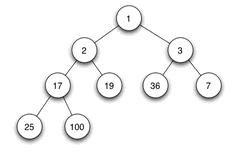（3）左右孩子：没有大小的顺序。

（4）堆的存储

（5）堆的操作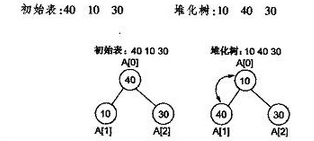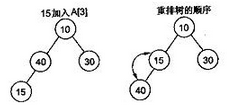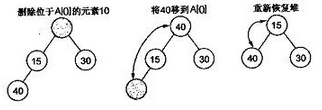//新加入i结点,其父结点为(i-1)/2
//参数：a：数组，i：新插入元素在数组中的下标
void minHeapFixUp(int a[], int i)
{
int j, temp;
temp = a[i];
j = (i-1)/2;      //父结点
while (j >= 0 && i != 0)
{
if (a[j] <= temp)//如果父节点不大于新插入的元素，停止寻找
break;
a[i]=a[j];     //把较大的子结点往下移动,替换它的子结点
i = j;
j = (i-1)/2;
}
a[i] = temp;
}  

//在最小堆中加入新的数据data
//a：数组，index：插入的下标，
void minHeapAddNumber(int a[], int index, int data)
{
a[index] = data;
minHeapFixUp(a, index);
} 

//a为数组，从index节点开始调整,len为节点总数 从0开始计算index节点的子节点为 2*index+1, 2*index+2,len/2-1为最后一个非叶子节点
void minHeapFixDown(int a[],int len,int index){
if(index>(len/2-1))//index为叶子节点不用调整
return;
int tmp=a[index];
int lastIndex=index;
while(index<=(len/2-1)){ //当下沉到叶子节点时，就不用调整了
if(a[2*index+1]<tmp) //如果左子节点大于该节点
lastIndex = 2*index+1;
//如果存在右子节点且大于左子节点和该节点
if(2*index+2<len && a[2*index+2]<a[2*index+1]&& a[2*index+2]<tmp)
lastIndex = 2*index+2;
if(lastIndex!=index){  //如果左右子节点有一个小于该节点则设置该节点的下沉位置
a[index]=a[lastIndex];
index=lastIndex;
}else break;  //否则该节点不用下沉调整
}
a[lastIndex]=tmp;//将该节点放到最后的位置
}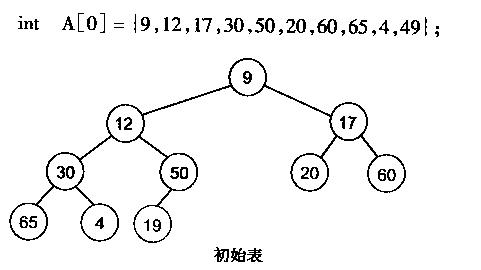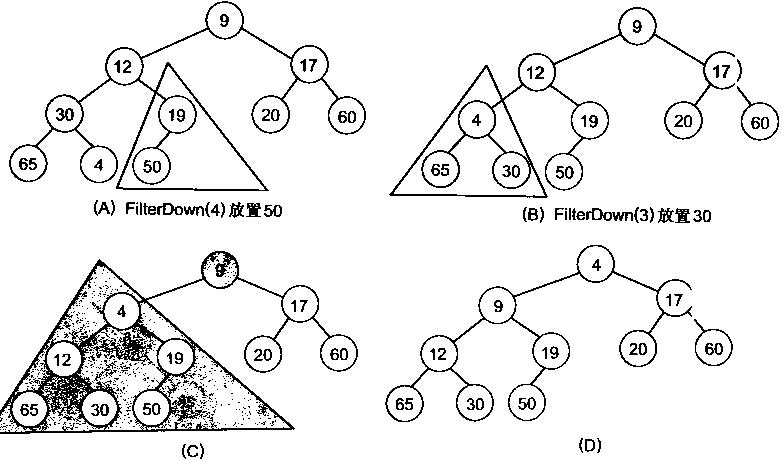//建立最小堆
//a:数组，n：数组长度
void makeMinHeap(int a[], int n)
{
for (int i = n/2-1; i >= 0; i--)
minHeapFixDown(a, i, n);
}  

（6）堆排序的实现

//array:待排序数组，len：数组长度
void heapSort(int array[],int len){
//建堆
makeMinHeap(array, len);
//根节点和最后一个叶子节点交换，并进行堆调整，交换的次数为len-1次
for(int i=0;i<len-1;++i){
//根节点和最后一个叶子节点交换
array += array[len-i-1];
array[len-i-1] = array-array[len-i-1];
array = array-array[len-i-1];

//堆调整
minHeapFixDown(array, 0, len-i-1);
}
}  

（7）堆排序的性能分析

# 2.5.1计数排序

（1）找出待排序的数组中最大的元素；
（2）统计数组中每个值为i的元素出现的次数，存入数组C的第i项；
（3）对所有的计数累加（从C中的第一个元素开始，每一项和前一项相加）；
（4）反向填充目标数组：将每个元素i放在新数组的第C(i)项，每放一个元素就将C(i)减去1。

#define MAXNUM 20    //待排序数的最大个数
#define MAX    100   //待排序数的最大值
int sorted_arr[MAXNUM]={0};

//计算排序
//arr:待排序数组，sorted_arr：排好序的数组，n：待排序数组长度
void countSort(int *arr, int *sorted_arr, int n)
{
int i;
int *count_arr = (int *)malloc(sizeof(int) * (MAX+1));

//初始化计数数组
memset(count_arr,0,sizeof(int) * (MAX+1));

//统计i的次数
for(i = 0;i<n;i++)
count_arr[arr[i]]++;
//对所有的计数累加，作用是统计arr数组值和小于小于arr数组值出现的个数
for(i = 1; i<=MAX; i++)
count_arr[i] += count_arr[i-1];
//逆向遍历源数组（保证稳定性），根据计数数组中对应的值填充到新的数组中
for(i = n-1; i>=0; i--)
{
//count_arr[arr[i]]表示arr数组中包括arr[i]和小于arr[i]的总数
sorted_arr[count_arr[arr[i]]-1] = arr[i];

//如果arr数组中有相同的数，arr[i]的下标减一
count_arr[arr[i]]--;
}
free(count_arr);
}

# 2.5.3桶排序

（1）设置一个定量的数组当作空桶子；
（2）寻访序列，并且把记录一个一个放到对应的桶子去；
（3）对每个不是空的桶子进行排序。
（4）从不是空的桶子里把项目再放回原来的序列中。

(1) 循环计算每个关键字的桶映射函数，这个时间复杂度是O(N)。

(2) 利用先进的比较排序算法对每个桶内的所有数据进行排序，对于N个待排数据，M个桶，平均每个桶[N/M]个数据，则桶内排序的时间复杂度为

typedef struct node
{
int keyNum;//桶中数的数量
int key;   //存储的元素
struct node * next;
}KeyNode;

//keys待排序数组，size数组长度，bucket_size桶的数量
void inc_sort(int keys[],int size,int bucket_size)
{
KeyNode* k=(KeyNode *)malloc(sizeof(KeyNode)); //用于控制打印
int i,j,b;
KeyNode **bucket_table=(KeyNode **)malloc(bucket_size*sizeof(KeyNode *));
for(i=0;i<bucket_size;i++)
{
bucket_table[i]=(KeyNode *)malloc(sizeof(KeyNode));
bucket_table[i]->keyNum=0;//记录当前桶中是否有数据
bucket_table[i]->key=0;   //记录当前桶中的数据
bucket_table[i]->next=NULL;
}

for(j=0;j<size;j++)
{
int index;
KeyNode *p;
KeyNode *node=(KeyNode *)malloc(sizeof(KeyNode));
node->key=keys[j];
node->next=NULL;

index=keys[j]/10;        //映射函数计算桶号
p=bucket_table[index];   //初始化P成为桶中数据链表的头指针
if(p->keyNum==0)//该桶中还没有数据
{
bucket_table[index]->next=node;
(bucket_table[index]->keyNum)++;  //桶的头结点记录桶内元素各数，此处加一
}
else//该桶中已有数据
{
//链表结构的插入排序
while(p->next!=NULL&&p->next->key<=node->key)
p=p->next;
node->next=p->next;
p->next=node;
(bucket_table[index]->keyNum)++;
}
}
//打印结果
for(b=0;b<bucket_size;b++)
//判断条件是跳过桶的头结点，桶的下个节点为元素节点不为空
for(k=bucket_table[b];k->next!=NULL;k=k->next)
{
printf("%d ",k->next->key);
}
}  

# 参考文献

计数排序、桶排序和基数排序.
 白话经典算法系列之六 快速排序 快速搞定.
白话经典算法系列之七 堆与堆排序.
算法与数据结构——C语言描述（第二版）.张乃孝
计数排序.百度百科

;